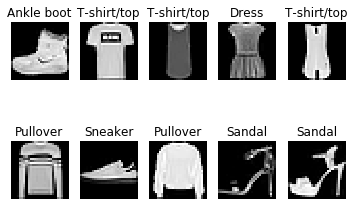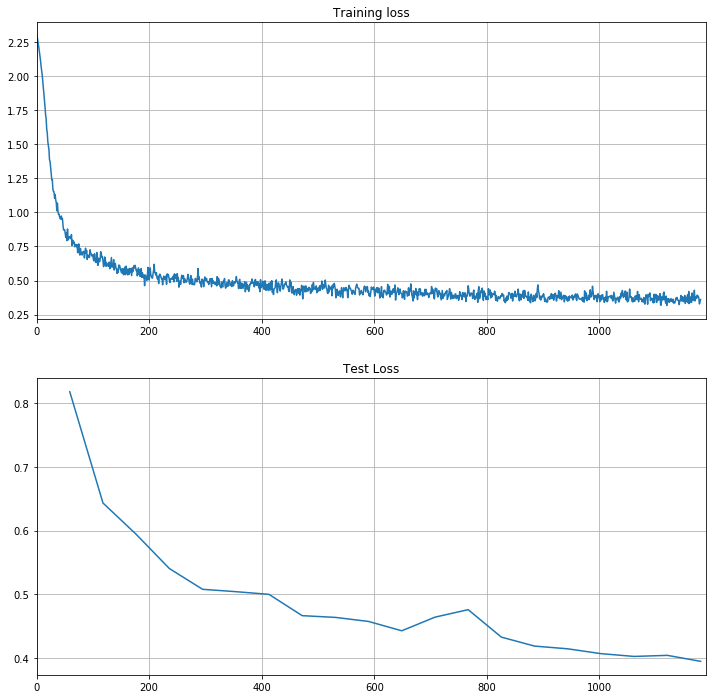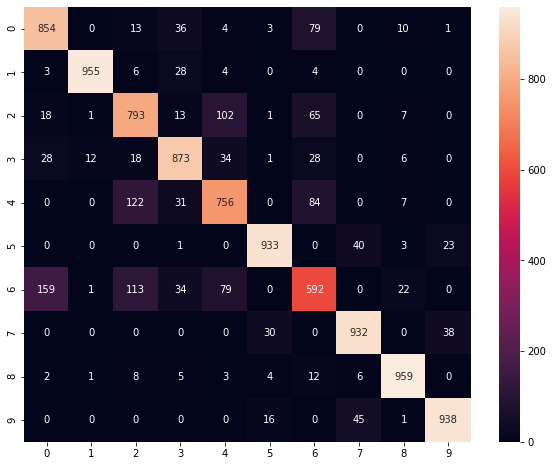# Fashion MNIST with BigDL¶

### AI and Predictive Analytics in Data-Center Environments - http://dcai.bsc.es¶

In this tutorial we will use BigDL to set up a Feed Forward neural network and use it to classify images from the Fashion-MNIST dataset. The Fashion-MNIST is a clothes-based version of the well-known MNIST handwritten digit database, with clothes instead of numbers. Let's start by importing the necessary packages, creating a Spark context and initializing the BigDL engine:

In :
import gzip
import numpy as np
import urllib.request
from pathlib import Path
import matplotlib.pyplot as plt
from bigdl.util.common import *
from bigdl.nn.layer import *
from bigdl.optim.optimizer import *
from bigdl.nn.criterion import *
from bigdl.optim.optimizer import *
import datetime as dt
from sklearn.metrics import confusion_matrix
from sklearn.metrics import accuracy_score
import pandas as pd
import seaborn as sns
from pyspark import SparkContext

# Get the spark context
sc = SparkContext.getOrCreate(conf=create_spark_conf().setMaster("local").set("spark.driver.memory","10g"))
init_engine()

Prepending /home/fjjm/.local/share/virtualenvs/exercises-oJA80Xyq/lib/python3.7/site-packages/bigdl/share/conf/spark-bigdl.conf to sys.path


Now we have to download the Fashion-MNIST data from its repository[https://github.com/zalandoresearch/fashion-mnist]. Here are a couple of functions to help us on that task (somewhat based on the _loadmnist function of the Fashion-MNIST repository):

In :
def download_mnist(path, kind='train'):
images_url = "http://fashion-mnist.s3-website.eu-central-1.amazonaws.com/{}-images-idx3-ubyte.gz".format(kind)
labels_url = "http://fashion-mnist.s3-website.eu-central-1.amazonaws.com/{}-labels-idx1-ubyte.gz".format(kind)

urllib.request.urlretrieve(images_url, os.path.join(path,"{}-images-idx3-ubyte.gz".format(kind)))
urllib.request.urlretrieve(labels_url, os.path.join(path,"{}-labels-idx1-ubyte.gz".format(kind)))
print("done.")

labels_path = os.path.join(path,"{}-labels-idx1-ubyte.gz".format(kind) )
images_path = os.path.join(path,"{}-images-idx3-ubyte.gz".format(kind) )

with gzip.open(labels_path, 'rb') as lbpath:

with gzip.open(images_path, 'rb') as imgpath:
images = np.frombuffer(imgpath.read(), dtype=np.uint8, offset=16).reshape(len(labels), 784)

return (images, labels)


We will download both the training and test data and save it into "bigdl-data/fashion_mnist":

In :
path = "bigdl-data/fashion_mnist"
Path(path).mkdir(parents=True, exist_ok=True)

Downloading the Fashion Mnist train dataset...done.


Before proceeding let's take a look at the data by showing the first 10 images together with their corresponding label:

In :
label_index = [ "T-shirt/top", "Trouser", "Pullover", "Dress", "Coat", "Sandal", "Shirt", "Sneaker", "Bag", "Ankle boot" ]

for i in range(10):
ax = plt.subplot(2, 5, i+1)
plt.imshow(np.column_stack(train_images[i].reshape(1, 28,28)),cmap="gray"); plt.axis("off")
ax.set_title(label_index[train_labels[i]])Now we need will load the data into RDDs and normalize it:

In :
training_mean = np.mean(train_images)
training_std = np.std(train_images)
rdd_train_images = sc.parallelize(train_images)
rdd_train_labels = sc.parallelize(train_labels)
rdd_test_images = sc.parallelize(test_images)
rdd_test_labels = sc.parallelize(test_labels)

train_feats = np.array([(feat - training_mean) / training_std for feat in train_images])
rdd_train_sample = sc.parallelize([Sample.from_ndarray(feat, label+1) for feat, label in zip(train_feats, train_labels)])

test_feats = np.array([(feat - training_mean) / training_std for feat in test_images])
rdd_test_sample = sc.parallelize([Sample.from_ndarray(feat, label+1) for feat, label in zip(test_feats, test_labels)])


We can also count the number of training and test samples to make sure we have the right amount:

In :
print("Number of training samples: {}".format(rdd_train_sample.count()))
print("Number of test samples: {}".format(rdd_test_sample.count()))

Number of training samples: 60000
Number of test samples: 10000


## Hyperparameter Setup¶

For the hyperparameter setup we will keep the same parameters as proposed in the BigDL tutorial notebooks for the MNIST dataset:

In :
learning_rate = 0.05
training_epochs = 20
batch_size = 1024
display_step = 1

# Network Parameters
n_hidden_1 = 256 # 1st layer number of features
n_hidden_2 = 256 # 2nd layer number of features
n_input = 784 # MNIST data input (img shape: 28*28)
n_classes = 10 # MNIST total classes (0-9 digits)


## The Model¶

Now we proceed to define the model, again following the structure proposed in the BigDL tutorial notebooks:

In :
from bigdl.nn.layer import *
model = Sequential()
# Hidden layer with ReLu activation
# Hidden layer with ReLu activation
# output layer
print(model)

creating: createSequential
creating: createReshape
creating: createLinear
creating: createReLU
creating: createLinear
creating: createReLU
creating: createLinear
creating: createLogSoftMax
Sequential[eae79e8c]{
[input -> (1) -> (2) -> (3) -> (4) -> (5) -> (6) -> (7) -> output]
(1): Reshape[edaf8670](784)
(2): Linear[mlp_fc1](784 -> 256)
(3): ReLU[4157b864](0.0, 0.0)
(4): Linear[mlp_fc2](256 -> 256)
(5): ReLU[b5920c60](0.0, 0.0)
(6): Linear[mlp_fc3](256 -> 10)
(7): LogSoftMax[36b24bb8]
}


Now we create the optimizer and set the validation logic

In :
from bigdl.optim.optimizer import *
from bigdl.nn.criterion import *
from bigdl.optim.optimizer import *
import datetime as dt

# Create an Optimizer
optimizer = Optimizer(
model=model,
training_rdd=rdd_train_sample,
criterion=ClassNLLCriterion(),
optim_method=SGD(learningrate=learning_rate),
end_trigger=MaxEpoch(training_epochs),
batch_size=batch_size)

# Set the validation logic
optimizer.set_validation(
batch_size=batch_size,
val_rdd=rdd_test_sample,
trigger=EveryEpoch(),
val_method=[Loss()]
)

app_name='multilayer_perceptron-'+dt.datetime.now().strftime("%Y%m%d-%H%M%S")
train_summary = TrainSummary(log_dir='/tmp/bigdl_summaries',
app_name=app_name)
train_summary.set_summary_trigger("Parameters", SeveralIteration(50))
val_summary = ValidationSummary(log_dir='/tmp/bigdl_summaries',
app_name=app_name)
optimizer.set_train_summary(train_summary)
optimizer.set_val_summary(val_summary)
print("saving logs to ",app_name)

creating: createClassNLLCriterion
creating: createDefault
creating: createSGD
creating: createMaxEpoch
creating: createDistriOptimizer
creating: createEveryEpoch
creating: createClassNLLCriterion
creating: createLoss
creating: createTrainSummary
creating: createSeveralIteration
creating: createValidationSummary
saving logs to  multilayer_perceptron-20200131-125811


Now that the setup is done, we can train the neural network by calling optimize():

In :
optimizer.optimize();


Once the network has been trained we can plot the loss on both the training and test dataset to see how it evolved during the training process:

In :
import matplotlib.pyplot as plt

plt.figure(figsize = (12,12))
plt.subplot(2,1,1)
plt.plot(loss_train[:,0],loss_train[:,1],label='Training loss')
plt.xlim(0,loss_train.shape+10)
plt.grid(True)
plt.title("Training loss")

plt.subplot(2,1,2)
plt.plot(loss_test[:,0],loss_test[:,1],label='Test loss')
plt.xlim(0,loss_train.shape+10)
plt.title("Test Loss")
plt.grid(True)Finally we can classify the images in the test dataset to see how good is our network at classifying clothes' pictures. Once we have done the classification we can check the prediction accuracy and the resulting confusion matrix:

In :
from sklearn.metrics import confusion_matrix
from sklearn.metrics import accuracy_score
import pandas as pd
import seaborn as sns

# Remember that labels are 1-indexed in BigDL:
y_pred = np.array(model.predict_class(rdd_test_sample).collect())-1
y_label = np.array([s.label.to_ndarray() - 1 for s in rdd_test_sample.collect()])

acc = accuracy_score(y_label, y_pred)
print("The prediction accuracy is %.2f%%"%(acc*100))

cm = confusion_matrix(y_label, y_pred)
df_cm = pd.DataFrame(cm)
plt.figure(figsize = (10,8))
sns.heatmap(df_cm, annot=True,fmt='d');

The prediction accuracy is 85.85%In [ ]: